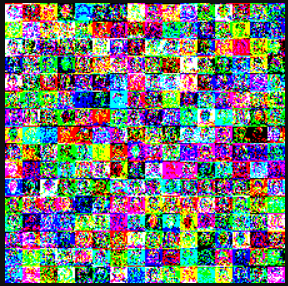# Restore the original image from ViT embedding

Let’s say I have an PIL Image of shape (224, 224, 3). I want to use Vision Transformer so that I can get the sequential embeddings of the image, which is of shape (1, 197, 768). Then, I’d like to restore the original image dimensions from the Tensor of shape (1, 197, 768), namely (224, 224, 3). I want every pixel to be at exactly the same position as they did in the original image. How do I achieve this? This is something I have tried:

``````em = res.squeeze()  # (1, 197, 768) -> (197, 768)
cls_token, emb = em, em[1:]  # exclude the class token
emb = emb.detach().numpy()  # convert torch.Tensor to numpy.ndarray
einops.rearrange(emb, '(l1 l2) (c h w) -> (h l1) (w l2) c', l2=14, h=16, c=3)  # use einops' operation
``````

The result: